# Understanding Parameter Uncertainty

Camera calibration generally aims to fit a suitable mathematical model to data in order to geometrically characterize one or more camera/lens-combinations.

Many users aim to lower the re-projection error (RPE) as much as possible. While this is, indeed, desirable in most cases, we advocate to judge calibration quality on more than the obtained RPE. First and foremost, parameter uncertainties play a crucial role for the camera system’s real world performance.

Let's dive deeper into this topic using a simpler data-fitting example which should unveil the importance of uncertainty in data fitting.

### A Fitting Problem

In the below figure, we have plotted a single sinusoidal half-wave which serves as our ground truth function that we aim to describe as faithfully as possible. (Note that in contrast to a camera model which maps $R^3 \rightarrow R^2$, this function is just $R \rightarrow R$, but the concepts apply irregardly.)Unfortunately, we do not know this real function in a calibration setting. Instead we would have a number of sampled values which are affected by noise and possibly bias. In camera calibration the sources of noise and bias include sensor dark current, shot noise, quantisation noise, defocus, etc. Below we have sampled data with noise distributed according to the normal distribution $\mathcal{N}\left(0, 0.05 \right)$.In this case, we have 8 data points and our aim is to fit a function, which hopefully resembles the true underlying function as good as possible. For technical reasons, we might only have samples from the central part of the domain, which in this case is the interval $[0; \pi]$. This is a very typical case also in camera calibration, when e.g. chessboard detection might require that the entire target is inside the image, leaving the outer image regions un- or under-sampled.

### Parabolic Fit

An easy model to fit using ordinary least squares would be a parabola (a 2nd degree polynomial) $y = a x^2 + b x + c$. In the figure below the best fit parabola is shown, along with the fit's $95\%$ confidence bands.It can be observed that the true underlying model was reconstructed reasonably, but especially at the domain boundaries, where no data is given, the true function actually falls outside of the confidence region. Further, the confidence region has considerable width over the entire function meaning that a new sampling of data points will likely lead to a parabola with quite different coefficients.

We have computed a root-mean-square error (RMSE) of $0.026$, which is the root of the mean of the squares of the vertical red error-bars. This is again analogous to camera calibration, where usually the RMS of reprojection errors is reported.

The found best-fit parabola is:

$y = -0.12 x^2 + 1.42 x - 0.46$

We are also able to estimate the covariance of the paramers at our solution:

$\left[\begin{array}{*{3}c} C_{a,a} & C_{a,b} & C_{a,c} \\ C_{a,b} & C_{b,b} & C_{b,c} \\ C_{a,c} & C_{b,c} & C_{c,c} \\ \end{array}\right]$ = $\left[\begin{array}{*{3}c} 0.0036 & -0.0048 & 0.0014 \\ -0.0048 & 0.0074 & -0.0023 \\ 0.0014 & -0.0023 & 0.0007 \\ \end{array}\right]$

Square roots of the diagonal entries give us the standard deviation of each of the model parameters:

$\sigma_a = \sqrt{0.0036} = 0.06$

$\sigma_b = \sqrt{0.0074} = 0.08$

$\sigma_c = \sqrt{0.0007} = 0.03$

This tells us that there is considerable uncertainty in all of the parameters. Off-diagonal entries are also non-negligible which means that certain combinations of parameters have high uncertainty. Taking $C_{a,b} = -0.0048$ as an example, increasing $a$ a little while decreasing $b$ a little would yield a very similar RMSE value.

Note that the correlation $\rho(i, j) = C_{i,j}/(\sqrt{C_{i,i}} \sqrt{C_{j,j}})$ between parameters might be easier to interpret as it normalizes these values to between $-1$ and $1$.

### Increasing Model Flexibility

In our hunt for low RPE values, we might consider increasing the degree of the fitting polynomial. This would correspond to adding more parameters to our model (think $k_3, k_4, k_5, ...$ in the OpenCV distortion model). Below is a 5th-order polynomial fit along with its $95\%$ confidence band.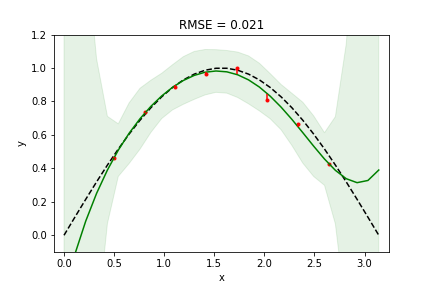Indeed we achieved a lower RPE value (from $0.026$ down to $0.021$), which a more flexible model would most always yield. However, at the right domain boundary we see our model diverting a lot from the truth. In addition, confidence bands are much wider, so we can't expect very consistent results when re-sampling. In statistics, this situation is called overfitting - there is a discrepancy between the model's flexibility and the amount of data to constraint the fit. In fact, since our noise has a standard deviation of $0.05$, that is the RMSE that we should expect to achieve.

In this case, the found polynomial coefficients (along with standard errors) are:

$a: -0.351 \pm 1.134$

$b: 2.399 \pm 4.911$

$c: -2.050 \pm 7.688$

$d: 1.253 \pm 5.539$

$e: -0.473 \pm 1.863$

$f: -0.067 \pm 0.237$

Standard errors are very high and many of these parameters are also heavily correlated. This gives rise to the very wide confidence bands above.

• Increasing model flexibility is not always warranted. Beware of over-fitting.
• Judging the fit's quality by its RMSE alone is not sufficient.

### Increasing the amount of data

With the last result in mind, one approach to avoid the overfitting problem could be to sample more data. In camera calibration terms, the number of target observations could be increased, or a calibration target with more visual features be used.

Below we have increased the number of samples to 50.We notice that confidence in the model has indeed increased significantly, but only in the region supported by data. RMSE has actually increased, but it was unnaturally low before due to the overfitting situation.

• Overfitting can be combated/avoided by sampling enough data.
• Higher-order polynomial models can "explode" outside regions with data support. (OpenCV users: beware of the higher-order polynomial distortion coefficients $k_2, k_3, k_4, ...$.)

We still have low confidence in our model near the domain boundaries, so let's revert to the 2nd-order polynomial (parabolic fit) and investigate what happens if a few data points are added in these regions: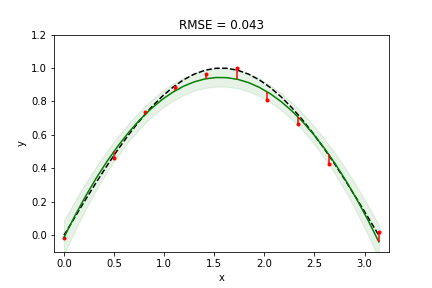RMSE has increased, but our fit is actually quite good across the entire domain.

• Samples from domain boundary regions help to constraint the model well.

### Modifying the model

So far, we have looked at polynomial models. Perhaps another mathematical model is better at describing the data. It might also work better in those regions where we do not have any information.

Below we have switched to a sinusoidal function $a \cdot \sin \left( w \cdot x \right ) + o$. Since we know that the true model is sinusoidal in nature, this model should fit really well.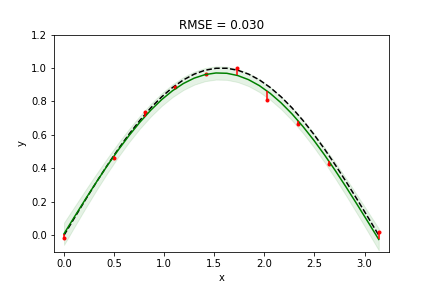And indeed it does. A result quite close to the ground truth with narrow confidence regions, even with comparatively little data.

Combining more data sampling and good coverage should yield even better results. Below we use the same sinusoidal model, but sample 50 values evenly spaced across the domain:By sampling enough data from the entire domain and choosing a function that is most capable of modelling the underlying true function with few parameters, we are able to achieve a very accurate and robust result.

Let's plot the obtained RMSE as a function of the number of samples (spaced uniformly on the interval $[0; \pi]$ with noise distribution $\mathcal{N}\left(0, 0.05 \right)$).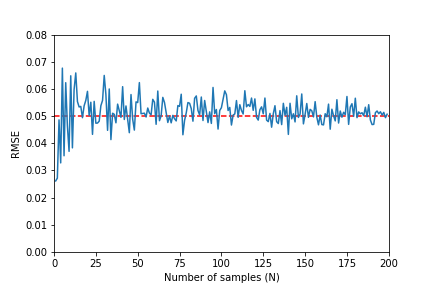As predicted, RMSE converges to the standard deviation of our the noise. A value clearly below it does not represent a good fit, but rather an overfitting situation.

### What This Means for Camera Calibration

Above we have explored a $R \rightarrow R$ function, as we can easily plot it and investigate different fits. However, everything that holds for data fitting here also applies to camera calibration which is just a higher dimensional data fitting problem.

In camera calibration we must also use the most suitable matematical model and use enough data to make all parameters well determined with as little standard error as possible. In addition, covariances/correlations should also be low. Similar to how confidence bands aid in the interpretation of covariance, we can propagate the uncertainty in calibration parameters to the image plane and visualize 95% confidence ellipses on the image plane. The screenshot below shows how Calibrator visualizes this result. The software also reports the RMS of simulated errors, which gives us an unbiased estimate of the true validation error.Taking things one step further, we might be interested in what the given uncertainty means for the precision of point triangulation in a multi-camera system. This given an easy to interpret value in units of meter/millimeters and reflects the benchmark that most user actually care about. Below is the "Triangulation error" view of Calibrator.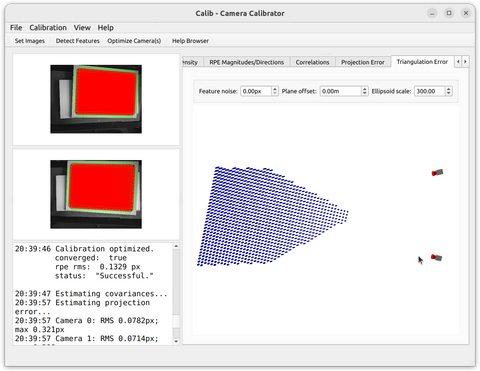To summarize:

• RMSE values should never stand alone as calibration success criteria.
• Using fewer parameter in the camera model and including more observations aids in avoiding overfitting.
• Confidence regions for individual parameters and their propagation into e.g. triangulation are important measures of calibration quality.

Calib.io's Camera Calibrator application lets you investigate all aspects of camera calibration errors and uncertainties. Standard errors, parameter covariances and triangulation errors are the camera calibration equivalents of confidence bands. It is crucial to take all these aspects into account for accurate camera calibration.

Thanks for helping us improve camera calibrations world wide. Your questions and comments are appreciated.

## 1 comment

• How is the simulated error of the RMS values calculated? Is it a cross validation-like estimation?

Nicholas Califano